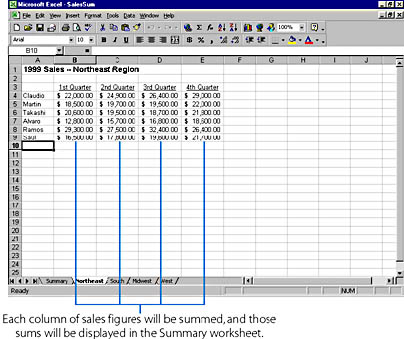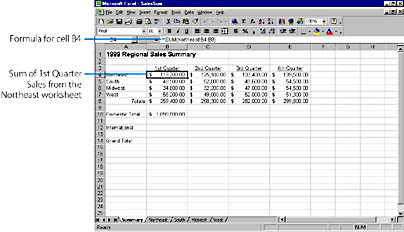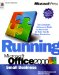# Linking Information Between Worksheets

When you create a workbook containing several worksheets, you'll often want to reference the data in one worksheet when you build a formula in another worksheet. Setting up a connection between worksheets is called creating a link in Excel terminology. For example, if your workbook contains a separate worksheet for each sales region in the country, you could create a Summary worksheet that includes sales data from each of the supporting worksheets. Linked worksheets also provide an additional advantage: When you change the source worksheet, Excel updates the related information in the linked worksheet.

To learn how to link worksheets together in different workbooks, see "Linking Information Between Workbooks."

The following procedure shows you how to create formulas that link worksheets together. The workbook used as an example contains five worksheet tabs, named Summary, Northwest, South, Midwest, and West. The four regional worksheets contain quarterly sales data for each of a company's sales representatives active in the region. (The sales reps are listed individually by name.) The Summary worksheet presents an overview of the sales activity throughout the year and uses several SUM formulas to calculate the quarterly totals from each of the linked worksheets. You might want to use this worksheet structure in your own workbooks.

To create formulas that calculate totals from linked worksheets and place them on a single summary worksheet, follow these steps. Our example uses regional sales data.

1. Create your regional worksheets in a workbook, or use your own data containing a similar pattern of detail-level worksheets that you want to sum up in a summary worksheet.
2. The following screen shows the sample worksheet named Northeast, containing quarterly sales figures for the six sales reps active in the Northeast sales region.ON THE WEB
The SalesSum.xls example is on the Running Office 2000 Reader's Corner page. For information about connecting to this Web site, read the Introduction.

3. Add a Summary worksheet to your workbook to display the totals from the other worksheets. (Add the worksheet by choosing Worksheet from the Insert menu, and then change the name to Summary or another appropriate name.)
4. Using the SUM function, add formulas to the Summary worksheet that compute totals. Begin each formula by typing =SUM(
5. To specify a range for the SUM function, click the worksheet tab you want to include in the formula, and then select the range of cells you want to use within the link. For example, to add the six sales figures from the 1st Quarter column in the Northeast worksheet, click the Northeast worksheet tab, and then select cells B4 through B9. The customized formula appears in the formula bar; the worksheet name and cell range are separated by an exclamation mark (Northeast!B4:B9).
6. Press the Enter key to complete the formula. Excel will add a closing parenthesis to complete the function. Excel calculates the result and displays it in cell B4 of the Summary worksheet, as shown in the following illustration. The completed formula also appears in the formula bar.
7.8. Repeat steps 2 through 5 to add linking formulas for the remaining summation cells.Running Microsoft Office 2000
ISBN: 1572319585
EAN: 2147483647
Year: 2005
Pages: 228